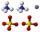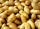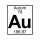# Density + comparing - math problems

#### Number of problems found: 6

• Sphere floatingWill float a hollow iron ball with an outer diameter d1 = 20cm and an inside diameter d2 = 19cm in the water? The iron density is 7.8 g/cm 3. (Instructions: Calculate the average sphere density and compare with the density of the water. )
• WeightThe kilogram weight has a volume of 116 ml. From which material is it probably made? Use substance density tables, e. G. mathematical-physical-chemical tables.
• Fe vs. H2OThe volume of what the body of the same weight is greater - iron or water?
• BeerWhich beer is better to drink: small beer (0.3 L) for 0.67 € or large (0.5 L) for 1.81 €?
• PotatoesCould 446 tons of potatoes (ρ = 771 kg/m³) fits in a warehouse with a volume of 699 m³ ?
• Unknown metalThe prism made of unknown metal has dimensions of 3 cm by 3 cm by 5 cm and a weight of 121 g. What metal is it? Write its chemical symbol.

We apologize, but in this category are not a lot of examples.
Do you have an interesting mathematical word problem that you can't solve it? Submit a math problem, and we can try to solve it.

We will send a solution to your e-mail address. Solved examples are also published here. Please enter the e-mail correctly and check whether you don't have a full mailbox.

Please do not submit problems from current active competitions such as Mathematical Olympiad, correspondence seminars etc...

Tip: Our Density units converter will help you with the conversion of density units. Density - math word problems. Comparing - math word problems.